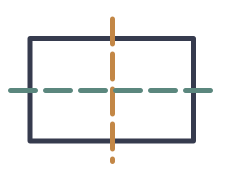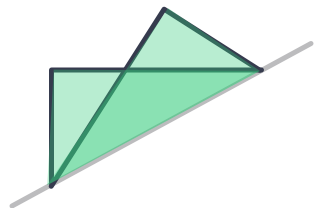Polygons with Symmetries

## Polygons with Symmetries

Polygons may have symmetry. Symmetry exists where part of a shape can be folded to overlap exacty the remaining part of the shape.A polygon may have one or more lines of symmetry. If the polygon was folded along a line of symmetry, then each half of the polygon would match exactly.A polygon may also have rotational symmetry. If the polygon is rotated about a point and it then looks like itself after being rotated, then it has rotational symmetry. The number of positions where this happens gives the Order of Rotational Symmetry: if it can be rotated three times to look like itself, then it has an Order of Rotational Symmetry of value 3.

A polygon with an order of rotational symmetry equal to 1 does not have rotational symmetry.## Example 1

How many lines of symmetry does a rectangle have?A rectangle has two lines of symmetry (it can be folded on two lines such that half of the shape can be matched to the other half).If the rectangle is reflected across the line of symmetry, then it does not match up onto the other side.